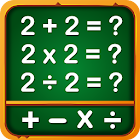Math Games, Learn Add, Subtract, Multiply & Divide

All Android applications categories

All Android games categories# Math Games, Learn Add, Subtract, Multiply & Divide

23.2K 9

9 Users
rating

## Screenshots

Description

#1 Math App on Google Play

Free Math Games for everyone from kids to adults. Best maths practice game to train your brain & is designed for all ages including kids, girls and boys, adults including parents and grandparents. Smallest in size Maths App on Google Play ! Easiest multiplication and division games with Addition and Subtraction games all in one app. Increase your brain power with an excellent educational game for learning mathematics for kids and adults of all ages

Languages: English, Spanish, German, Italian, Portuguese, French, Russian

Features :
- Subtraction games: Subtracting numbers to solve the equations
- Multiplication games: Multiplication tables learning and duel play mode
- Division games: Practice and learn Division tables
- Exponential and square root: counting math puzzles for kids and adults
- Multiply number game
- Math puzzle games
- Maths Times Tables

Each category has different play modes to improve math skills - Play, Learn, Quiz, Practice, Duel and Test. Math games can be Educational Learning for kids or brain training app for Adults. Basic and simple Math Game of addition, subtraction, multiplication and division with colorful worksheets. Each set of worksheet shows a score after completion.

Mathematical calculations to play and practice with simple addition, subtraction, multiplication and division. Now download and play for free on android ! Improve your mathematics skills or learn counting numbers. The games are so simple and easy even the youngest kids can play it.

Fun addition and subtraction games with multiplication tables, designed for all smartphones and tablets, no internet connection needed. Including Times Tables Multiplications and Division. With this educational app, parents, teachers and educators can help children to learn faster. This Math Genius game is a fun game designed mainly around the topics of addition, subtraction, multiplication and division